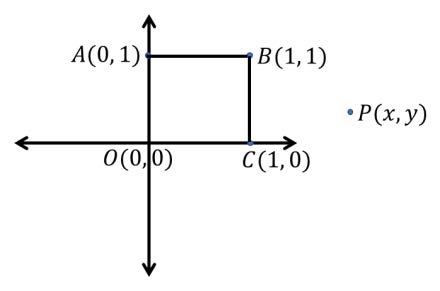# The sum of the square of the distance of a point from (0, 0), (0, 1), (1, 1), (1, 0) is 18 and its locus is a circle. If d is the diameter of the circle, then find d2

Solution:(OP) 2 + (AP) 2 + (PC) 2 + (PB) 2=18

=> x2 + y2 + x2 + (y – 1) 2 + (x – 1) 2 + y2 + (x – 1) 2 + (y – 1) 2 =18

=> 4x2 + 4y2 – 4x – 4y=14

=> x2 + y2 – x – y=7/2

=> r=2

=> d = 4 => d2 = 16(0)(0)